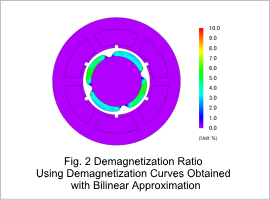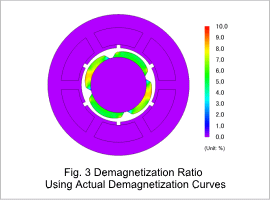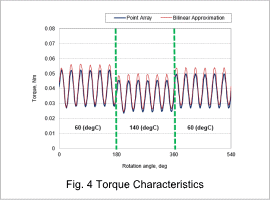## [JAC274] SPM Motor Thermal Demagnetization Analysis Using Bonded NdFeB Magnets

Remember me

*It is different from the service for JMAG WEB MEMBER (free member). Please be careful.
About authentication ID for JMAG website

### Overview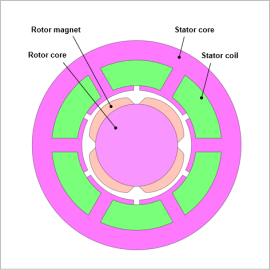Because SPM motors are, due to their structure, succeptible to demagnetizing fields from rotor magnets, it is necessary to consider the influence of magnet demagnetization on motor characteristics.
In order to properly grasp this demagnetization, the demagnetizing characteristics that magnets possess should be correctly accounted for. When there exists magnetization characteristics that make it difficult to observe knickpoints on demagnetization curves like those of bonded NdFeB bonded magnets, it becomes difficult to recreate demagnetization curves with linear approximation. By correctly expressing demagnetization curves using point arrays, the influence of demagnetization on various characteristics can be accounted for at a high level of accuracy.
In this example, an SPM motor thermal demagnetization analysis is performed using bonded NdFeB magnets. Both the influence on torque waveforms when changing rotor magnet temperatures and the distribution of the demagnetization ratio within the magnets are evaluated.

### Demagnetization Curves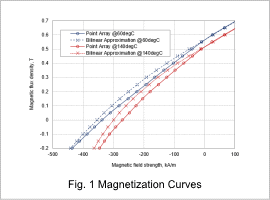Fig. 1 shows the demagnetization curves of the rotor magnets used in this document. The blue lines show characteristics at 60 deg C, and the red lines show characteristics at 140 deg C. The dashed lines are the demagnetization curves obtained with bilinear approximation, and the solid lines are the actual demagnetization curves plotted with point arrays.
It can be observed that differences exists in the demagnetization curves obtained with bilinear approximation compared to the actual demagnetization curves. From this, it can be considered that a difference in results occurs when accounting for thermal demagnetization using the two types of demagnetization curves shown.[/jmaglightbox]

### Effect on Motor Characteristics

The influence on torque characteristics was evaluated when magnet temperatures were changed from 60 deg C to 140 deg C and back to 60 deg C again when running at an excitation condition of a phase current amplitude of 5 A at an angle 80 deg.
Fig. 2 and Fig. 3 shows the demagnetization ratio of the rotor magnets after irreversible demagnetization with contours. The demagnetization ratio is defined as the amount of variation for magnet residual magnetic flux density prior to additional load and heating. It is understood that a difference occurs between the bilinear approximation of the demagnetization curves and using the actual demagnetization curves.
Fig. 4 shows torque characteristics, where it understood that differences in torque characteristics occur. Compared to the average torque after irreversible demagnetization, it is understood that there is an error of 8 % that occurs when using the bilinear approximation demagnetization curves.
This shows that demagnetization can be correctly accounted for by specifying demagnetization curves with arbitrary point arrays when using magnets where knickpoints on demagnetization curves are difficult to observe.